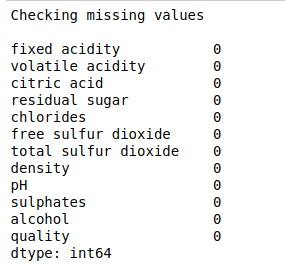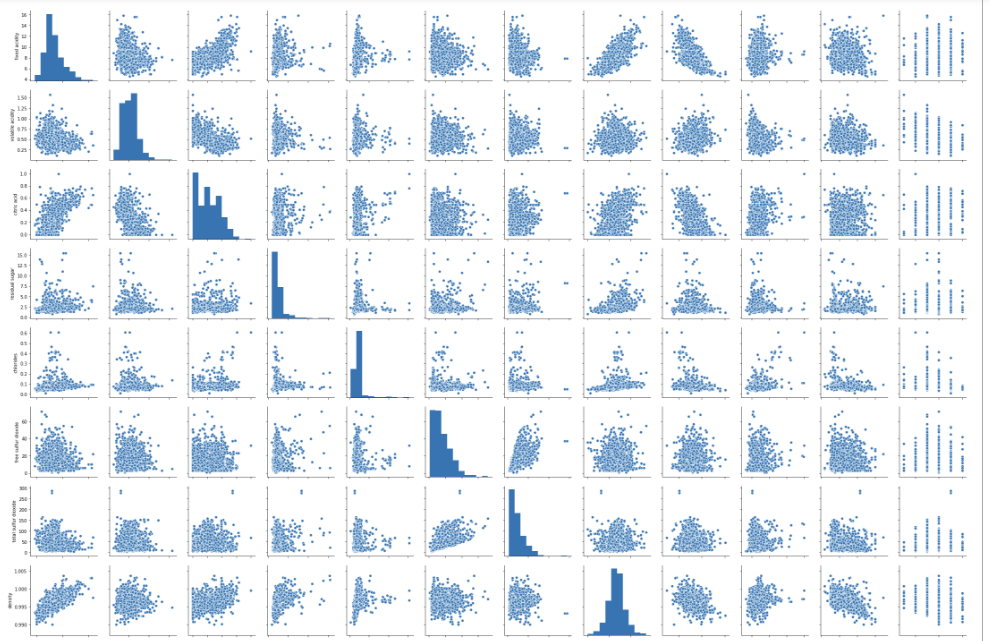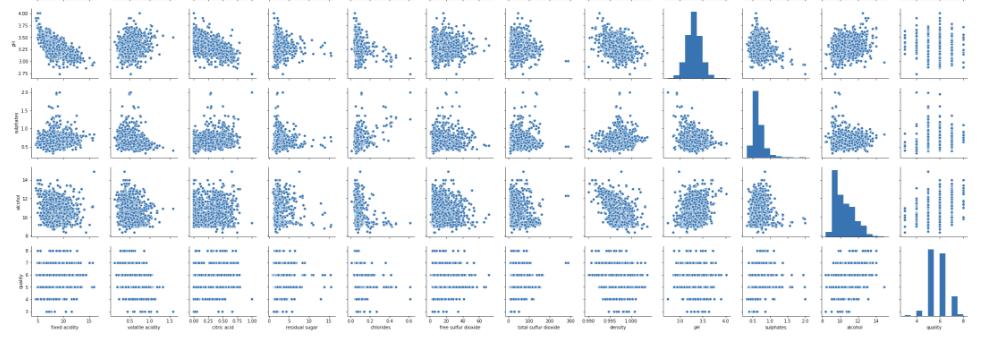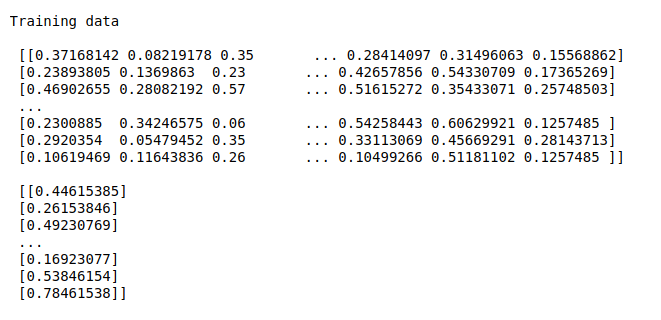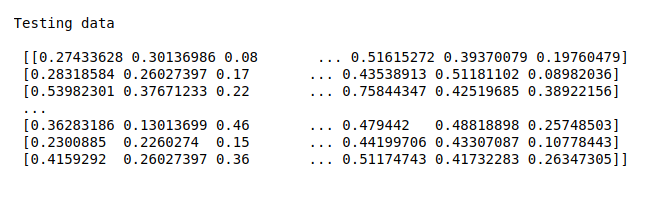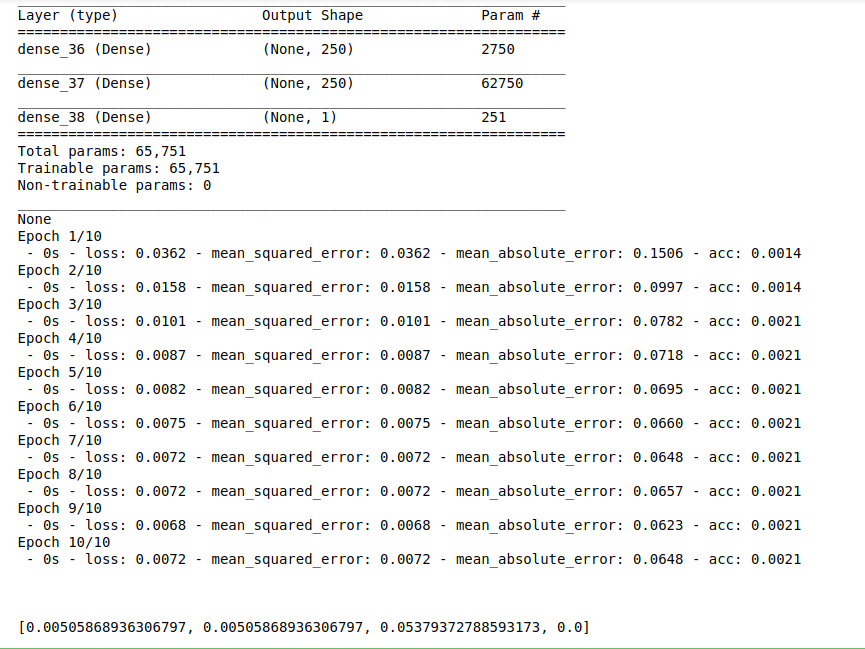• #5, First Floor, 4th Street Dr. Subbarayan Nagar Kodambakkam, Chennai-600 024 Landmark : Samiyar Madam
• pro@slogix.in
• +91- 81240 01111

### How to predict alcohol level in wine data set using DNN model from keras in python?

###### Description

To build a DNN model for predict the alcohol level in wine data set using keras.

###### Input

Wine quality data set.

MSE
MAE
Accuracy score

#### process:

Define the independent and dependent variable..

Build the deep neural networks.

Initiate activation and optimizer functions according to the problem.

Split the data into train and testing set.

Fit the training set into the model.

Predict the test results using DNN.

Calculate the MSE, MAE and accuracy.

###### Sample Code

#import necessary libraries
import warnings
warnings.filterwarnings(“ignore”)
import pandas as pd
import numpy as np
import matplotlib.pyplot as plt
import seaborn as sns
from keras.models import Sequential
from keras.layers import Dense
from sklearn.model_selection import train_test_split

#Make it as a data frame
df = pd.DataFrame(data)

#Checking missing values
print(“Checking missing values\n”)
print(df.isnull().sum())

#pairplot for input variables
sns.pairplot(df)
plt.show()

#Features selection
X = data.iloc[:,0:10]
y = data.iloc[:,10].values

#Pre processing of data
from sklearn.preprocessing import MinMaxScaler
sc= MinMaxScaler()
X= sc.fit_transform(X)
y= y.reshape(-1,1)
y=sc.fit_transform(y)

#Split the data into train and testing
X_train, X_test, Y_train, Y_test = train_test_split(X, y, test_size=0.1, random_state=42)

#Print training data
print(“Training data\n\n”,X_train,”\n\n”,Y_train)
print(“\n\n”)

#Print testing data
print(“Testing data\n\n”,X_test)
print(“\n\n”)

#Create DNN model for regression
dim = X_train.shape
model = Sequential()
print(model.summary())

#Compile the model

#Here we train the Network.
model.fit(X_train, Y_train,batch_size=30, epochs = 10, verbose = 2)

#Here we evaluate the model
results = model.evaluate(X_test,Y_test,verbose = 2,batch_size = 30)
print(“\n\n”)
print(results)

###### Screenshots